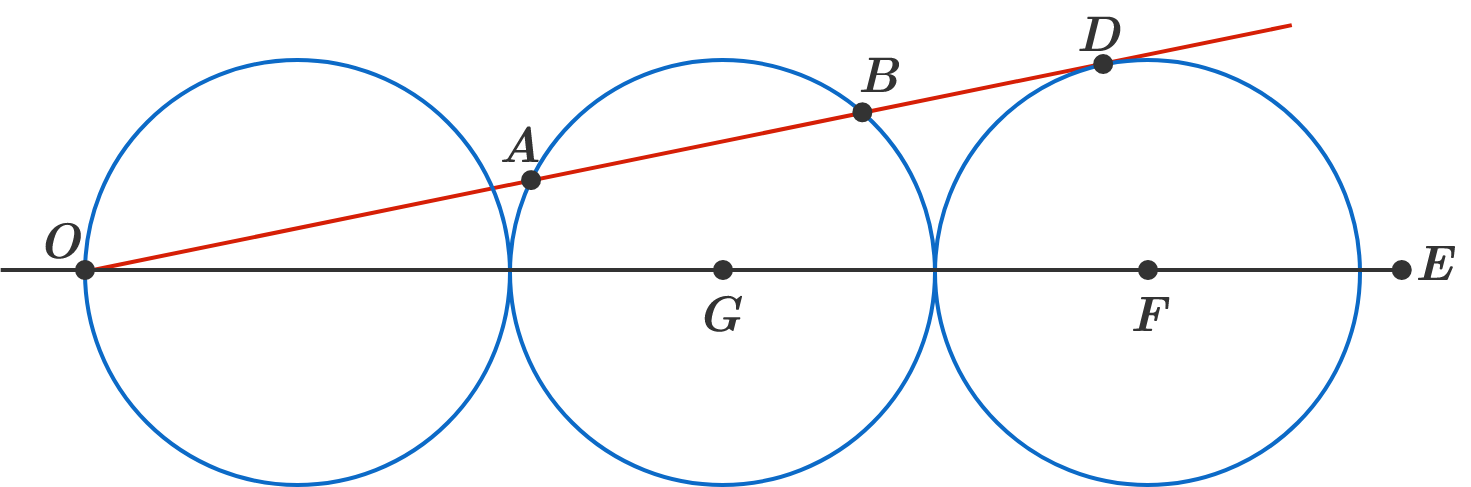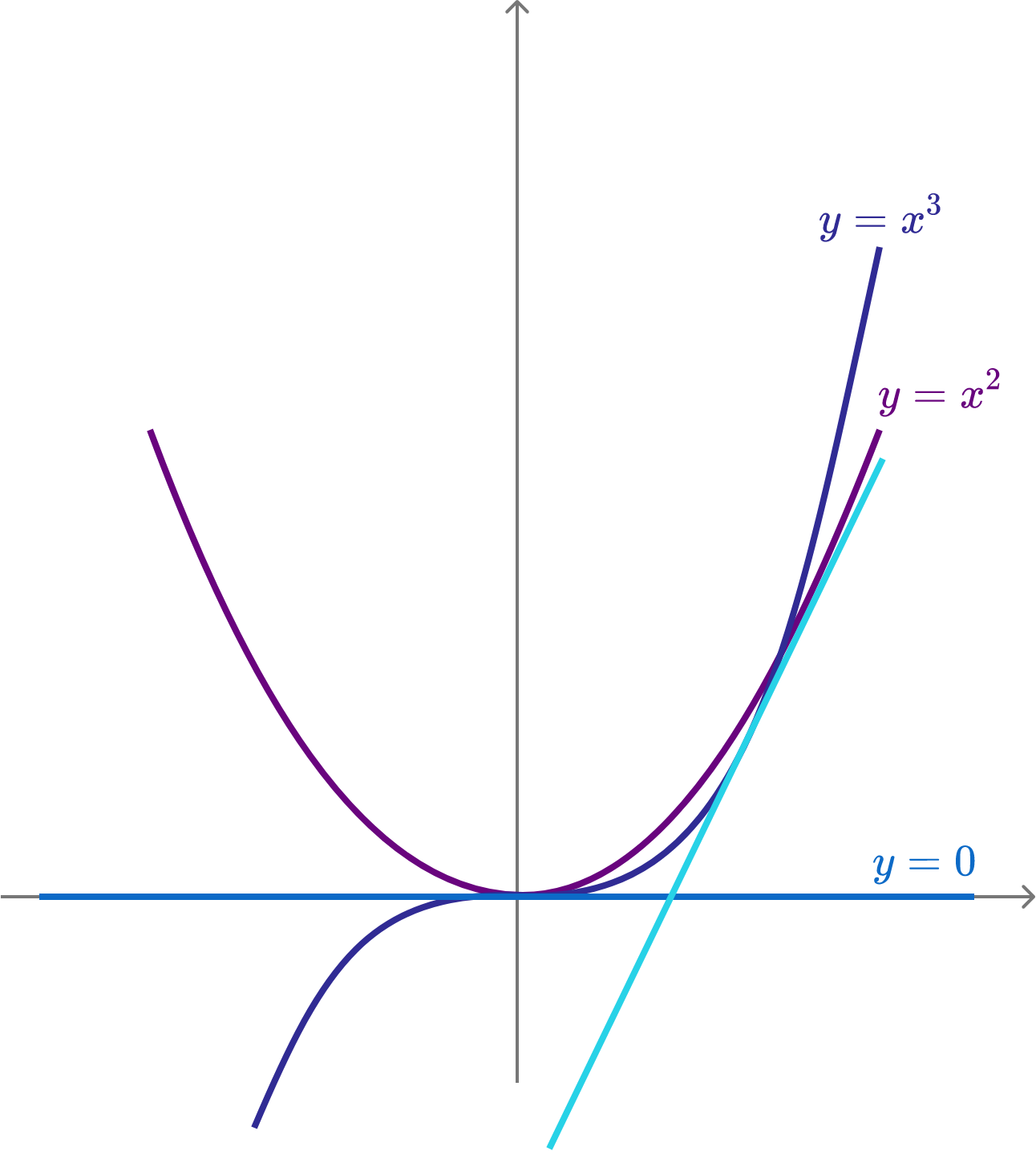# Problems of the Week

Contribute a problem

# 2018-12-03 Intermediate

If Earth somehow contracted to $\frac {1}{8 }$ of its current volume instantly (through some magical internal forces), while keeping its shape and mass, then how long would a day be?Three unit circles are drawn tangent to each other with all their centers lying on line segment $OE.$

From $O,$ line segment $OD$ is drawn such that it's tangent to the rightmost circle.

If the length of the chord $AB$ is $\frac ab$, where $a$ and $b$ are coprime positive integers, then what is $a+b?$Note: I did not create this problem; I simply solved it and decided to post it on here. Credit goes to my math teacher.

After paying for a newspaper with 4 quarters, the newspaper came out. But, to my surprise, my 4 quarters also came back out because the machine was broken. I quickly took note of how I paid for the paper: "4 quarters." After spending over a quarter of an hour at the machine, I paid for a newspaper in every possible way.

How many newspapers did I end up with?

Assumptions:

• Only 4 kinds of coins can be used: pennies ($.01), nickels ($.05), dimes ($.10), and quarters ($.25).
• The order of the coins placed into the machine doesn't matter.

The 3 little pigs have recently established their own construction company.

1. The eldest pig has a daily wage of $350 and builds a house in 9 days. 2. The middle pig has a daily wage of$250 and builds a house in 12 days.
3. The youngest pig has a daily wage of $150 and builds a house in 16 days. Their new client is the wolf, now a real estate entrepreneur. The wolf would like to contract the pigs to build a house. The pigs provide the following conditions on their work: • Exactly 2 pigs work on the house each day (so that the other pig can call for help if something happens). • Each pig must have at least 1 day off during this working period. • If the job is done before the end of the last day, the wolf is still charged a full-day rate for that day. Under the 3 pigs' conditions, what is the least amount of money (in$) the wolf can invest to complete this project?The $x$-axis is a common tangent of the two curves $y = x^{2}$ and $y = x^{3}$.

Suppose the slope of the other common tangent (in sky blue) of these two curves is expressed as $\frac{p}{q},$ where $p$ and $q$ are coprime positive integers.

What is $p+q?$

×